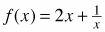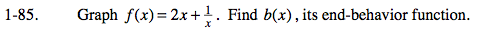### Home > CALC > Chapter 1 > Lesson 1.2.5 > Problem1-85

1-85.

Graph. Find b(x), its end-behavior function. 1-85 HW eTool (Desmos). Homework Help ✎When LOOKING for the end behavior of a graph, it is important to zoom out. Ignoring holes and asymptotes, does the function look like it is linear? quadratic? neither?

If the function has an asymptote, then the asymptote is its end behavior.

$f(x)=2x+\frac{1}{x}\text{ is written in a form that makes it easy to see the equation of the end behavior. }$

$\text{Think about what happens to the }\frac{1}{x}\text{ part of } f(x)\text{ when the function is evaluated at very high or very }$

$\text{low values... As }x\rightarrow \pm \infty ,\frac{1}{x}\rightarrow 0.$

Therefore, the end behavior of f(x) is y = 2x + 0 or y = 2x.

Use the eTool below to view the graph and the end behavior function.
Click the link at right for the full version of the eTool: Calc 1-85 HW eTool